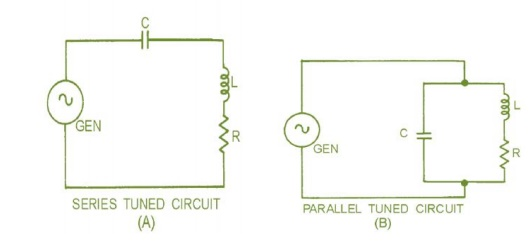Home | | Electronic Circuits II | Resonance circuits

# Resonance circuits

The frequency applied to an LCR circuit causes XL and XC to be equal, and the circuit is RESONANT.

Resonance circuits

The frequency applied to an LCR circuit causes XL and XC to be equal, and the circuit is RESONANT. If XL and XC are equal ONLY at one frequency (the resonant frequency). This fact is the principle that enables tuned circuits in the radio receiver to select one particular frequency and reject all others.

This is the reason why so much emphasis is placed on XL and X C . figure 1-1 Shows that a basic tuned circuit consists of a coil and a capacitor, connected either in series, view (A), or in parallel, view (B). The resistance (R) in the circuit is usually limited to the inherent resistance of the components (particularly the resistance of the coil).Tuned amplifier

ü   Communication circuit widely uses tuned amplifier and they are used in MW & SW radio frequency 550 KHz – 16 MHz, 54 – 88 MHz, FM 88 – 108 MHz, cell phones 470 - 990 MHz

ü   Band width is 3 dB frequency interval of pass band and –30 dB frequency interval

ü   Tune amplifiers are also classified as A, B, C similar to power amplifiers based on conduction angle of devices.

Series resonant circuit

Series resonant features minimum impedance (RS) at resonant.

ü   f r = ½ √LC; q = L/Rs at resonance L=1/c, BW=fr/Q

ü   It behaves as purely resistance at resonance, capacitive below and inductive above resonance

Paralel resonant circuit

ü   Paralel resonance features maximum impedance at resonance = L/RsC

ü   At resonance Fr=1/2√1/(LC-Rs2/L2); if Rs=0, fr=1/2√(LC)

ü   At resonance it exhibits pure resistance and  below fr parallel circuit exhibits inductive and above capacitive impedance

Study Material, Lecturing Notes, Assignment, Reference, Wiki description explanation, brief detail
Electronic Circuits : Tuned Amplifiers : Resonance circuits |